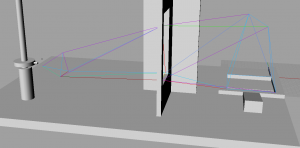# Joshua

## 06 Feb 2013A sketch I did of the truss in Rhino. The truss must reach the cylindrical pole to lift a load, and only touch the playing field at the opposite end. The colored lines are the springs of the truss. Using Grasshopper I simulated the springs and color coded each line according to whether it was in tension(reds and purples) or compression (greens) or unstressed (light blue).

The idea is to find the best (optimal) truss given a bunch of constraints and a specific loading.  The truss is a mesh where each node is a connection between struts, and each edge is a strut, which is approximated as an ideal elastic spring. This is actually an assignment for a mechE class called Stress Analysis, but the nature of the project makes it perfect for creating a genetic algorithm for optimizing the truss.

The basic structure would be:

1)mate parent trusses two create a bunch of offspring.

2)Introduce mutations.

3)Test all offspring for fitness.

4)Select fittest offspring.

5)Repeat until solution appears to be converging (not changing very much compared to the previous solution).

The main goal would be to  minimize deflection and weight of the truss.

In general optimization problems have a ‘cost function’ or a function that evaluates how good or bad a certain combination of variables is.  For example a chair could have a few parameters like height, weight, softness, and some complex mathematical expression might describe how these parameters combine to make a comfortable or not-so-comfortable chair.  In the case of a truss, where any number of connections are possible and the variables involve a large number of vertices and edges, determining such a cost function would be incredibly difficult.   Instead my plan is to simulate the behavior of the truss with springs under an idealized load.  The performance of the truss (like deflection, or maximum stress) would be quantified and used directly as the fitness of that truss. Thus the cost function would instead be a physical simulation.  Run times may be an issue.

I was trying to do this in processing but realized that it really is not made for computing precise values.  So I will do this in Matlab.